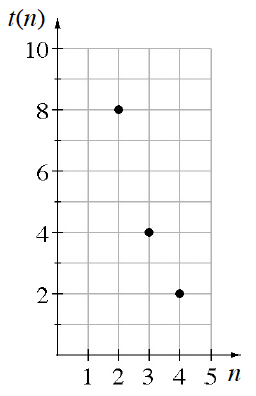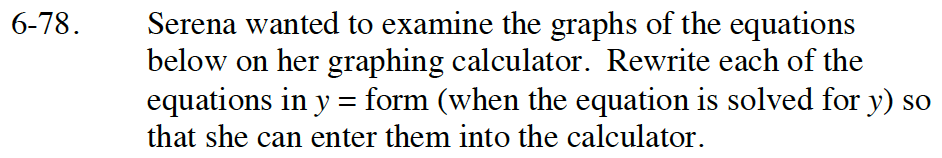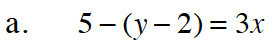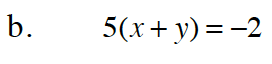Home > GB8I > Chapter cc46 > Lesson cc46.2.3 > Problem6-78

6-78.
1.6-78. Serena wanted to examine the graphs of the equations below on her graphing calculator. Rewrite each of the equations in y = form (when the equation is solved for y) so that she can enter them into the calculator. 6-78 HW eTool (Desmos). Homework Help ✎

1. 5 −(y − 2) = 3x

2. 5(x + y) = −2Subtract 5 from both sides of the equation.

−(y − 2) = 3x − 5

Multiply both sides by −1.

y − 2 = −3x + 5

Now finish solving for y by adding 2 to both sides.

y = −3x + 7Multiply (x + y) by 5.

Use the eTool below to view the graphs of the equations.
Click on the link at right for the full eTool version: Int1 6-78 HW eTool.